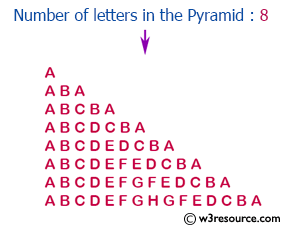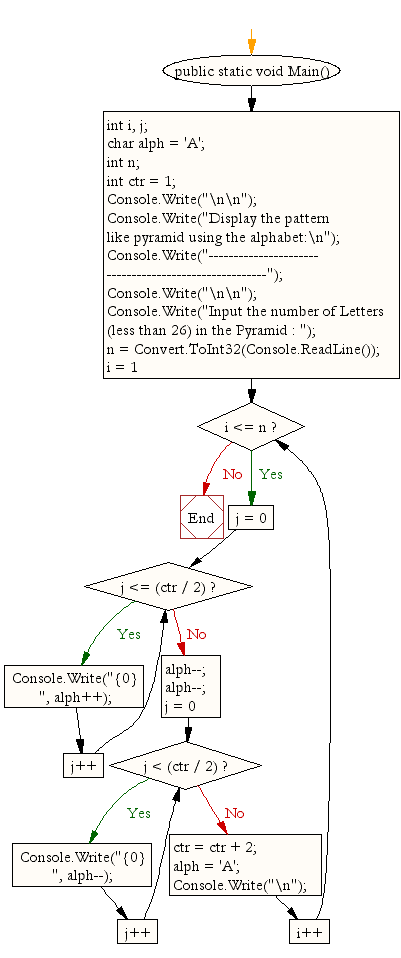﻿ C Sharp - Show a pattern like pyramid using the alphabet# C# Sharp Exercises: Display the pattern like pyramid using the alphabet

## C# Sharp For Loop: Exercise-40 with Solution

Write a C# Sharp Program to display the following pattern using the alphabet.
The pattern is as follows:

```A
A B A
A B C B A
A B C D C B A
```

Pictorial Presentation:Sample Solution:

C# Sharp Code:

``````using System;
public class Exercise40
{
public static void Main()
{
int i, j;
char alph = 'A';
int n;
int ctr = 1;

Console.Write("\n\n");
Console.Write("Display the pattern like pyramid using the alphabet:\n");
Console.Write("------------------------------------------------------");
Console.Write("\n\n");

Console.Write("Input the number of Letters (less than 26) in the Pyramid : \n");

for (i = 1; i <= n; i++) {
for (j = 0; j <= (ctr / 2); j++)
{
Console.Write("{0} ", alph++);
}
alph--;
alph--;

for (j = 0; j < (ctr / 2); j++) {
Console.Write("{0} ", alph--);
}
ctr = ctr + 2;
alph = 'A';
Console.Write("\n");
}
}
}
```
```

Sample Output:

```Display the pattern like pyramid using the alphabet:
------------------------------------------------------
Input the number of Letters (less than 26) in the Pyramid : 8
A
A B A
A B C B A
A B C D C B A
A B C D E D C B A
A B C D E F E D C B A
A B C D E F G F E D C B A
A B C D E F G H G F E D C B A
```

Flowchart:C# Sharp Code Editor:

Contribute your code and comments through Disqus.

What is the difficulty level of this exercise?

Test your Programming skills with w3resource's quiz.

﻿